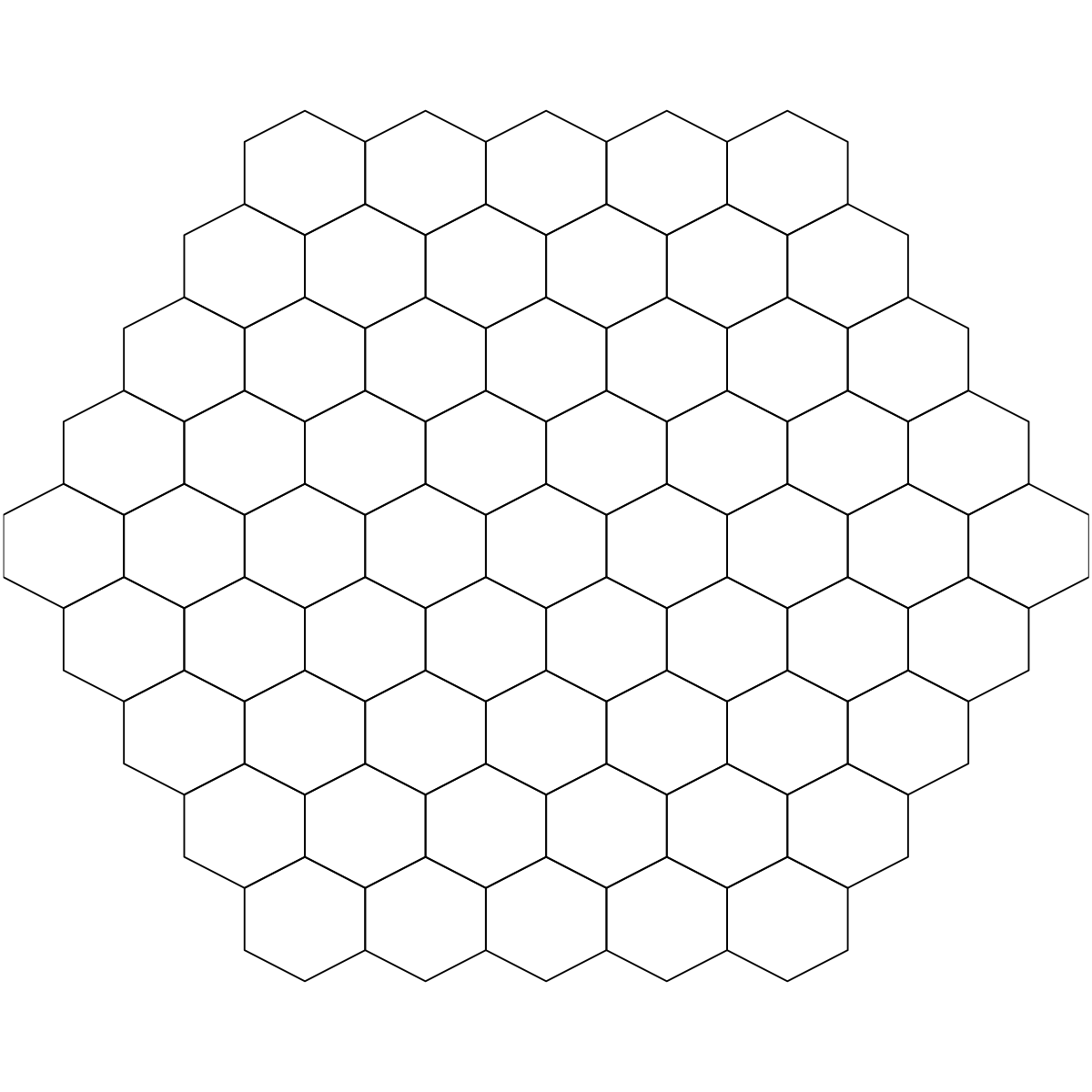## Function to define the topology of a map grid

### Description

`sTopology` is supposed to define the topology of a 2D map grid. The topological shape can be either a supra-hexagonal grid or a hexagonal/rectangle sheet. It returns an object of "sTopol" class, containing: the total number of hexagons/rectangles in the grid, the grid xy-dimensions, the grid lattice, the grid shape, and the 2D coordinates of all hexagons/rectangles in the grid. The 2D coordinates can be directly used to measure distances between any pair of lattice hexagons/rectangles.

### Usage

```sTopology(data = NULL, xdim = NULL, ydim = NULL, nHex = NULL, lattice = c("hexa",
"rect"), shape = c("suprahex", "sheet", "triangle", "diamond", "hourglass", "trefoil",
"ladder", "butterfly", "ring", "bridge"), scale = 5)```

### Arguments

data
a data frame or matrix of input data
xdim
an integer specifying x-dimension of the grid
ydim
an integer specifying y-dimension of the grid
nHex
the number of hexagons/rectangles in the grid
lattice
the grid lattice, either "hexa" for a hexagon or "rect" for a rectangle
shape
the grid shape, either "suprahex" for a supra-hexagonal grid or "sheet" for a hexagonal/rectangle sheet. Also supported are suprahex's variants (including "triangle" for the triangle-shaped variant, "diamond" for the diamond-shaped variant, "hourglass" for the hourglass-shaped variant, "trefoil" for the trefoil-shaped variant, "ladder" for the ladder-shaped variant, "butterfly" for the butterfly-shaped variant, "ring" for the ring-shaped variant, and "bridge" for the bridge-shaped variant)
scale
the scaling factor. Only used when automatically estimating the grid dimension from input data matrix. By default, it is 5 (big map). Other suggested values: 1 for small map, and 3 for median map

### Value

an object of class "sTopol", a list with following components:

• `nHex`: the total number of hexagons/rectanges in the grid. It is not always the same as the input nHex (if any); see "Note" below for the explaination
• `xdim`: x-dimension of the grid
• `ydim`: y-dimension of the grid
• `r`: the hypothetical radius of the grid
• `lattice`: the grid lattice
• `shape`: the grid shape
• `coord`: a matrix of nHex x 2, with each row corresponding to the coordinates of a hexagon/rectangle in the 2D map grid
• `call`: the call that produced this result

### Note

The output of nHex depends on the input arguments and grid shape:

• How the input parameters are used to determine nHex is taken priority in the following order: "xdim & ydim" > "nHex" > "data"
• If both of xdim and ydim are given, `nHex=xdim*ydim` for the "sheet" shape, `r=(min(xdim,ydim)+1)/2` for the "suprahex" shape
• If only data is input, `nHex=scale*sqrt(dlen)`, where dlen is the number of rows of the input data, and scale can be 5 (big map), 3 (median map) and 1 (normal map)
• With nHex in hand, it depends on the grid shape:
• For "sheet" shape, xy-dimensions of sheet grid is determined according to the square root of the two biggest eigenvalues of the input data
• For "suprahex" shape, see `sHexGrid` for calculating the grid radius r. The xdim (and ydim) is related to r via `xdim=2*r-1`

### Examples

```# For "suprahex" shape
sTopol <- sTopology(xdim=3, ydim=3, lattice="hexa", shape="suprahex")

# Error: "The suprahex shape grid only allows for hexagonal lattice"
# sTopol <- sTopology(xdim=3, ydim=3, lattice="rect", shape="suprahex")

# For "sheet" shape with hexagonal lattice
sTopol <- sTopology(xdim=3, ydim=3, lattice="hexa", shape="sheet")

# For "sheet" shape with rectangle lattice
sTopol <- sTopology(xdim=3, ydim=3, lattice="rect", shape="sheet")

# By default, nHex=19 (i.e., r=3; xdim=ydim=5) for "suprahex" shape
sTopol <- sTopology(shape="suprahex")

Warning message:
Ignore the input parameters but use the default radius.

# By default, xdim=ydim=5 (i.e., nHex=25) for "sheet" shape
sTopol <- sTopology(shape="sheet")

# Determine the topolopy of a supra-hexagonal grid based on input data
# 1) generate an iid normal random matrix of 100x10
data <- matrix(rnorm(100*10,mean=0,sd=1), nrow=100, ncol=10)
# 2) from this input matrix, determine nHex=5*sqrt(nrow(data))=50,
# but it returns nHex=61, via "sHexGrid(nHex=50)", to make sure a supra-hexagonal grid
sTopol <- sTopology(data=data, lattice="hexa", shape="suprahex")
# sTopol <- sTopology(data=data, lattice="hexa", shape="trefoil")

# do visualisation
visHexMapping(sTopol,mappingType="indexes")

library(ggplot2)# another way to do visualisation
df_polygon <- sHexPolygon(sTopol)
df_coord <- data.frame(sTopol\$coord, index=1:nrow(sTopol\$coord))
gp <- ggplot(data=df_polygon, aes(x,y,group=index)) +
geom_polygon(aes(fill=factor(stepCentroid%%2))) +
coord_fixed(ratio=1) + theme_void() + theme(legend.position="none") +
geom_text(data=df_coord, aes(x,y,label=index), color="white")
```

## Source code

`sTopology.r`

## Source man

`sTopology.Rd` `sTopology.pdf`

`sHexGrid`, `visHexMapping`